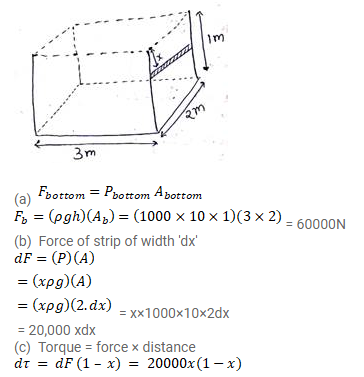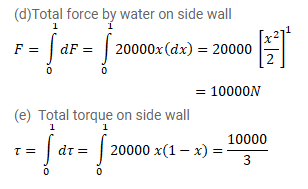# Water is filled in a rectangular tank of size 3m × 2m × 1m.

Question:

Water is filled in a rectangular tank of size $3 m \times 2 m \times 1 m$.

(a) Find the total force exerted by the water on the bottom surface of the tank.

(b) Consider a vertical side of area $2 \mathrm{~m} \times 1 \mathrm{~m}$. Take a horizontal strip of width $\partial \mathrm{x}$ metre in this side, situated at a depth of $x$ metre from the surface of water. Find the force by the water on strip.

(c) Find the torque of the force calculated in part (b) about the bottom edge of this side.

(d) Find the total force by the water on this side.

(c) Find the total torque by the water on this side about the bottom edge. Neglect the atmospheric pressure and take $g=$ $10 \mathrm{~m} / \mathrm{s}^{2}$.

Solution: